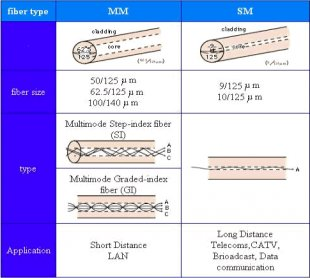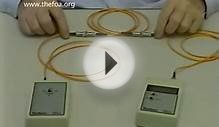# Fiber Optic cable loss

July 4, 2017This document is a quick reference to some of the formulas and important information related to optical technologies. This document focuses on decibels (dB), decibels per milliwatt (dBm), attenuation and measurements, and provides an introduction to optical fibers.

There are no specific requirements for this document.

This document is not restricted to specific software and hardware versions.

The information in this document was created from the devices in a specific lab environment. All of the devices used in this document started with a cleared (default) configuration. If your network is live, make sure that you understand the potential impact of any command.

A decibel (dB) is a unit used to express relative differences in signal strength. A decibel is expressed as the base 10 logarithm of the ratio of the power of two signals, as shown here:

dB = 10 x Log10 (P1/P2)

where Log10 is the base 10 logarithm, and P1 and P2 are the powers to be compared.

Note: Log10 is different from the Neparian Logarithm (Ln or LN) base e logarithm.

You can also express signal amplitude in dB. Power is proportional to the square of the amplitude of a signal. Therefore, dB is expressed as:

dB = 20 x Log10 (V1/V2)

where V1 and V2 are the amplitudes to be compared.

1 Bell (not currently used) = Log10 (P1/P2)

1 decibel (dB) = 1 Bell / 10 = 10 * Log10 (P1/P2)

dBr = dB (relative) = dB = 10 * Log10 (P1/P2)

• Log10 (AxB) = Log10 (A) + Log10 (B)
• Log10 (A/B) = Log10 (A) - Log10 (B)
• Log10 (1/A) = - Log10 (A)
• Log10 (0, 01) = - Log10 (100) = -2
• Log10 (0, 1) = - Log10(10) = - 1
• Log10 (1) = 0
• Log10 (2) = 0, 3
• Log10 (4) = 0, 6
• Log10 (10) = 1
• Log10 (20) = 1, 3

Log10 (2 x 10) = Log10 (2) + Log10 (10) = 1 + 0, 3

• Log10 (100) = 2
• Log10 (1000) = 3
• Log10 (10000) = 4

This table lists the Logarithm and dB (decibel) power ratios:

Power Ratio dB = 10 x Log10 (Power Ratio)
AxB x dB = 10 x Log10(A) + 10 x Log10(B)
A/B x dB = 10 x Log10(A) - 10 x Log10(B)
1/A x dB = + 10 x Log10 (1/A) = - 10 x Log10 (A)
0, 01 - 20 dB = - 10 x Log10(100)
0, 1 - 10 dB = 10 x Log10 (1)
0 dB = 10 x Log10 (1)
3 dB = 10 x Log10 (2)
6 dB = 10 x Log10 (4)
10 10 dB = 10 x Log10 (10)
20 13 dB = 10 x (Log10 (10) + Log10 (2))
100 20 dB = 10 x Log10 (100)
1000 30 dB = 10 x Log10 (1000)
10000 40 dB = 10 x Log10 (10000)

dBm = dB milliwatt = 10 x Log10 (Power in mW / 1 mW)

Power Ratio dBm = 10 x Log10 (Power in mW / 1 mW)
1 mW 1 mW/1mW=1 0 dBm = 10 x Log10 (1)
2 mW 2 mW/1mW=2 3 dBm = 10 x Log10 (2)
4 mW 4 mW/1mW=4 6 dBm = 10 x Log10 (4)
10 mW 10 mW/1mW=10 10 dBm = 10 x Log10 (10)
0, 1 W 100 mW/1mW=100 20 dBm = 10 x Log10 (100)
1 W 1000 mW/1mW=1000 30 dBm = 10 x Log10 (1000)
10 W 10000mW/1mW=10000 40 dBm = 10 x Log10 (10000)

dBW = dB Watt = 10 x Log10 (Power in W / 1 W)

1 W / 1 W = 1 0 dBW = 10 x Log10 (1)
2 W 2 W / 1 W = 2 3 dBW = 10 x Log10 (2)
4 W 4 W / 1 W = 4 6 dBW = 10 x Log10 (4)
10 W / 1 W = 10 10 dBW = 10 x Log10 (10)
100 mW 0, 1 W / 1 W = 0, 1 -10 dBW = -10 x Log10 (10)
0, 01 W / 1 W = 1/100 -20 dBW = -10 x Log10 (100)
0, 001W/1W=1/1000 -30 dBW = -10 x Log10 (1000)

This table compares the power and voltage gains:

dB Voltage Ratio
1, 00 10, 00 3, 16
1, 26 1, 12 11 12, 59 3, 55
1, 58 12 15, 85 3, 98
2, 00 1, 41 13 19, 95 4, 47
2, 51 14 25, 12 5, 01
1, 78 15 31, 62 5, 62
16 39, 81 6, 31
2, 24 17 50, 12 7, 08
18 63, 10 7, 94
2, 82 19 79, 43 8, 91
100, 00
##### YOU MIGHT ALSO LIKELoss-CalculationFiber Optic Cable: Insertion Loss TestingHow to Test db Loss of Multiomode Fiber Optic Cable